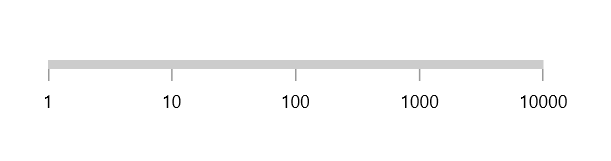# Axis in WinUI Linear Gauge

26 May 202122 minutes to read

The `Linear axis` is a linear scale, where a set of values can be plotted along with a linear or custom scale, based on the design requirements. Axis elements such as labels, ticks, and axis line can be easily customized with built-in properties.

## Axis customization

### Setting axis minimum and maximum value

The `Minimum` and `Maximum` properties of an axis can be used to customize the axis value range. The default value of `Minimum` is 0 and `Maximum` is 100.

``````<gauge:SfLinearGauge>
<gauge:SfLinearGauge.Axis>
<gauge:LinearAxis Minimum="-60"
Maximum="60" />
</gauge:SfLinearGauge.Axis>
</gauge:SfLinearGauge>``````
``````SfLinearGauge sfLinearGauge = new SfLinearGauge();
sfLinearGauge.Axis.Minimum = -60;
sfLinearGauge.Axis.Maximum = 60;
this.Content = sfLinearGauge;``````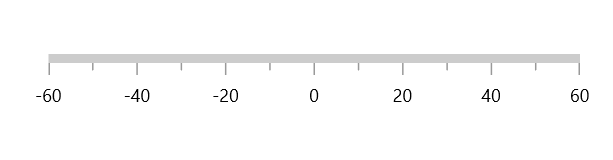### Interval

The interval between labels can be customized using the `Interval` property of axis.

``````<gauge:SfLinearGauge>
<gauge:SfLinearGauge.Axis>
<gauge:LinearAxis Interval="20" />
</gauge:SfLinearGauge.Axis>
</gauge:SfLinearGauge>``````
``````SfLinearGauge sfLinearGauge = new SfLinearGauge();
sfLinearGauge.Axis.Interval = 20;
this.Content = sfLinearGauge;``````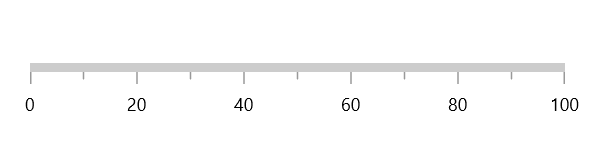### Axis direction customization

The direction of `Linear axis` can be customized by the `IsInversed` property of `Linear gauge`.

When the `IsInversed` property is true, the axis can be placed in right-to-left direction. When the `IsInversed` property is set to false, the axis will be positioned in left-to-right direction.

``<gauge:SfLinearGauge IsInversed="True" />``
``this.Content = new SfLinearGauge { IsInversed = true };``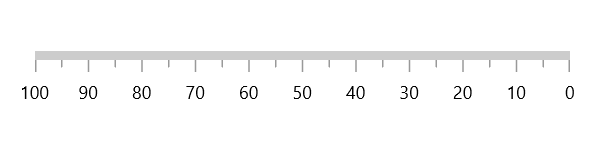### Mirrored axis support

`Linear axis` can be displayed as mirrored by using the `IsMirrored` property of `Linear gauge`.

When the `IsMirrored` property is true, the axis can be rendered in mirrored or opposite direction.

``<gauge:SfLinearGauge IsMirrored="True" />``
``this.Content = new SfLinearGauge { IsMirrored = true };``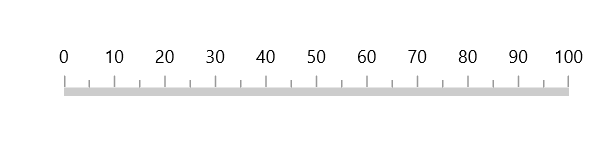### Axis orientation support

The orientation of `Linear axis` can be customized by using the `Orientation` property of `Linear gauge`.

When the `Orientation` property is `Vertical`, the axis will be rendered in bottom-to-top direction.

``<gauge:SfLinearGauge Orientation="Vertical" />``
``this.Content = new SfLinearGauge { Orientation = Orientation.Vertical };``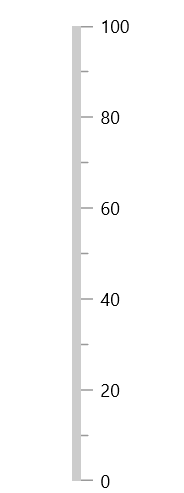### Maximum number of labels per 100 logical pixels

The maximum number of labels that should be present within 100 logical pixels length can be customized using the `MaximumLabelsCount` property of the axis. This property is applicable only for automatic range calculation and will not work, if you set value for interval property of an axis.

``````<gauge:SfLinearGauge>
<gauge:SfLinearGauge.Axis>
<gauge:LinearAxis MaximumLabelsCount="1" />
</gauge:SfLinearGauge.Axis>
</gauge:SfLinearGauge>``````
``````SfLinearGauge sfLinearGauge = new SfLinearGauge();
sfLinearGauge.Axis.MaximumLabelsCount = 1;
this.Content = sfLinearGauge;``````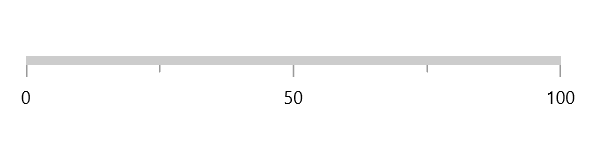## Axis line customization

The linear axis line can be customized using the following properties:

### Axis line stroke thickness

The axis line will be rendered based on the pixel value given in `AxisLineStrokeThickness`.

``````<gauge:SfLinearGauge>
<gauge:SfLinearGauge.Axis>
<gauge:LinearAxis AxisLineStrokeThickness="30" />
</gauge:SfLinearGauge.Axis>
</gauge:SfLinearGauge>``````
``````SfLinearGauge sfLinearGauge = new SfLinearGauge();
sfLinearGauge.Axis.AxisLineWidth = 30;
this.Content = sfLinearGauge;``````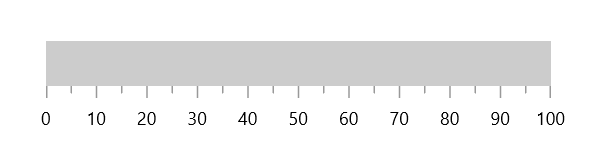### Setting axis line stroke color

To apply the color to the axis line using the `AxisLineStroke` property of linear axis.

``````<gauge:SfLinearGauge>
<gauge:SfLinearGauge.Axis>
<gauge:LinearAxis AxisLineFill="BlueViolet" />
</gauge:SfLinearGauge.Axis>
</gauge:SfLinearGauge>``````
``````SfLinearGauge sfLinearGauge = new SfLinearGauge();
sfLinearGauge.Axis.AxisLineFill = new SolidColorBrush(Colors.BlueViolet);
this.Content = sfLinearGauge;``````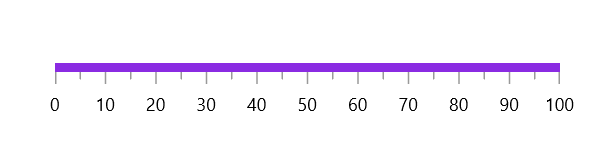### Axis line visibility

The visibility of the axis line can be customized using the `ShowAxisLine` property of axis. By default, this property is set to true.

``````<gauge:SfLinearGauge>
<gauge:SfLinearGauge.Axis>
<gauge:LinearAxis ShowAxisLine="False" />
</gauge:SfLinearGauge.Axis>
</gauge:SfLinearGauge>``````
``````SfLinearGauge sfLinearGauge = new SfLinearGauge();
sfLinearGauge.Axis.ShowAxisLine = false;
this.Content = sfLinearGauge;``````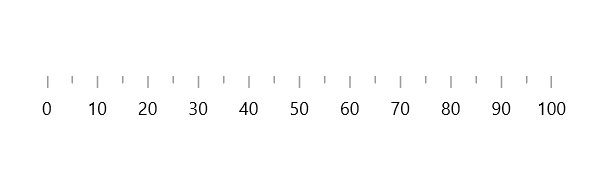## Axis label customization

The axis labels can be customized using the following properties:

• `Foreground` – Allows to customize the color of the labels.
• `FontFamily` – Allows to specify the font family for labels.
• `FontStyle` – Allows to specify the font style for labels.
• `FontWeight` – Allows to specify the font weight for labels.
• `FontSize` – Allows to specify the font size for labels.
``````<gauge:SfLinearGauge>
<gauge:SfLinearGauge.Axis>
<gauge:LinearAxis FontSize="15"
Foreground="Red"
FontFamily="Comic Sans MS"
FontWeight="Bold"
FontStyle="Italic" />
</gauge:SfLinearGauge.Axis>
</gauge:SfLinearGauge>``````
``````SfLinearGauge sfLinearGauge = new SfLinearGauge();
sfLinearGauge.Axis.FontSize = 15;
sfLinearGauge.Axis.Foreground = new SolidColorBrush(Colors.Red);
sfLinearGauge.Axis.FontFamily = new FontFamily("Comic Sans MS");
sfLinearGauge.Axis.FontWeight = FontWeights.SemiBold;
sfLinearGauge.Axis.FontStyle = Windows.UI.Text.FontStyle.Italic;
this.Content = sfLinearGauge;``````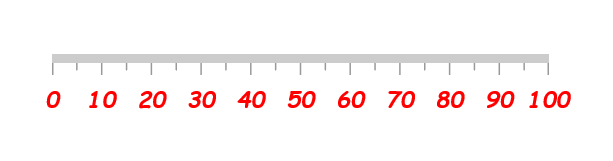### Formatting axis label

The `LabelFormat` allows to customize the axis label with the globalized label format.

``````<gauge:SfLinearGauge>
<gauge:SfLinearGauge.Axis>
<gauge:LinearAxis LabelFormat="c" />
</gauge:SfLinearGauge.Axis>
</gauge:SfLinearGauge>``````
``````SfLinearGauge sfLinearGauge = new SfLinearGauge();
sfLinearGauge.Axis.LabelFormat = "c";
this.Content = sfLinearGauge;``````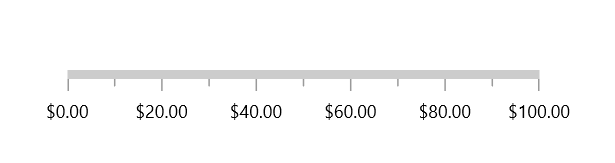### Template support for axis label

The `LabelTemplate` property allows you to define the customized style for the axis label as shown in the following code sample.

``````<Page.Resources>
<DataTemplate x:Key="labelTemplate">
<Border Background="Gray"
<TextBlock Text="{Binding Text}"
Foreground="White"
FontStyle="Normal"
FontWeight="Bold"
Margin="3" />
</Border>
</DataTemplate>
</Page.Resources>

<gauge:SfLinearGauge>
<gauge:SfLinearGauge.Axis>
<gauge:LinearAxis LabelTemplate="{StaticResource labelTemplate}" />
</gauge:SfLinearGauge.Axis>
</gauge:SfLinearGauge>``````
``````SfLinearGauge sfLinearGauge = new SfLinearGauge();
sfLinearGauge.Axis.LabelTemplate = this.Resources["labelTemplate"] as DataTemplate;
this.Content = sfLinearGauge;``````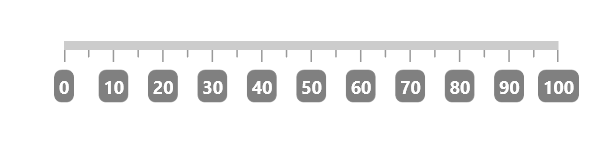### Label visibility

The `ShowLabels` property of axis allows to enable or disable the visibility of labels. The default value of this property is true.

``````<gauge:SfLinearGauge>
<gauge:SfLinearGauge.Axis>
<gauge:LinearAxis ShowLabels="False" />
</gauge:SfLinearGauge.Axis>
</gauge:SfLinearGauge>``````
``````SfLinearGauge sfLinearGauge = new SfLinearGauge();
sfLinearGauge.Axis.ShowLabels = false;
this.Content = sfLinearGauge;``````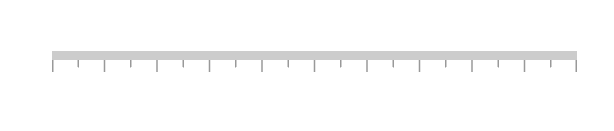### Label placement

The linear axis allows to position the labels either inside or outside of the axis line using the `LabelPosition` property. By default, labels are positioned inside the axis line.

``````<gauge:SfLinearGauge>
<gauge:SfLinearGauge.Axis>
<gauge:LinearAxis LabelPosition="Outside" />
</gauge:SfLinearGauge.Axis>
</gauge:SfLinearGauge>``````
``````SfLinearGauge sfLinearGauge = new SfLinearGauge();
sfLinearGauge.Axis.LabelPosition = GaugeLabelsPosition.Outside;
this.Content = sfLinearGauge;``````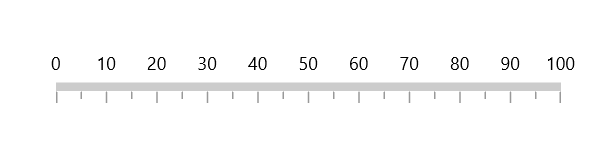### Label position customization

The `LabelOffset` property allows to adjust the distance between the axis line and the labels. By default, the value of the label offset is 5.

``````<gauge:SfLinearGauge>
<gauge:SfLinearGauge.Axis>
<gauge:LinearAxis LabelOffset="40" />
</gauge:SfLinearGauge.Axis>
</gauge:SfLinearGauge>``````
``````SfLinearGauge sfLinearGauge = new SfLinearGauge();
sfLinearGauge.Axis.LabelOffset = 40;
this.Content = sfLinearGauge;``````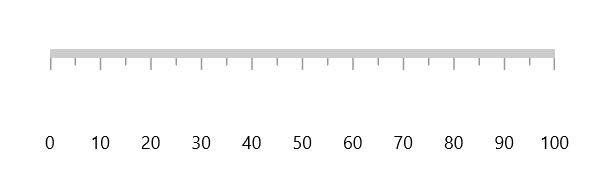## Tick customization

The major and minor tick lines of an axis can be customized using the following properties:

### Tick length

The major and minor tick lines will be rendered based on the pixel values given in `MajorTickLength` and `MinorTickLength`.

``````<gauge:SfLinearGauge>
<gauge:SfLinearGauge.Axis>
<gauge:LinearAxis MajorTickLength="15"
MinorTickLength="10" />
</gauge:SfLinearGauge.Axis>
</gauge:SfLinearGauge>``````
``````SfLinearGauge sfLinearGauge = new SfLinearGauge();
sfLinearGauge.Axis.MajorTickLength = 15;
sfLinearGauge.Axis.MinorTickLength = 10;
this.Content = sfLinearGauge;``````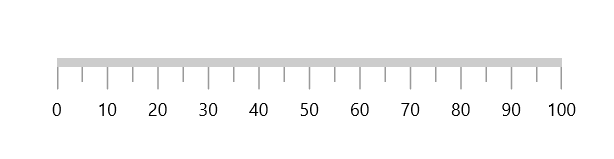### Setting style for major ticks

The `MajorTickStyle` property allows you to define the style for the major tick’s as shown in the following code example.

``````<Page.Resources>
<Style x:Key="MajorTickLineStyle"
TargetType="Line">
<Setter Property="Stroke"
Value="Black"></Setter>
<Setter Property="StrokeThickness"
Value="1.5"></Setter>
</Style>
</Page.Resources>

<gauge:SfLinearGauge>
<gauge:SfLinearGauge.Axis>
<gauge:LinearAxis MajorTickStyle="{StaticResource MajorTickLineStyle}" />
</gauge:SfLinearGauge.Axis>
</gauge:SfLinearGauge>``````
``````SfLinearGauge sfLinearGauge = new SfLinearGauge();
sfLinearGauge.Axis.MajorTickStyle = this.Resources["MajorTickLineStyle"] as Style;
this.Content = sfLinearGauge;``````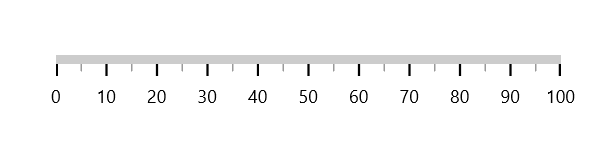### Setting style for minor ticks

The `MinorTickStyle` property allows you to define the style for the minor tick’s as shown in the following code example.

``````<Page.Resources>
<Style x:Key="MinorTickLineStyle"
TargetType="Line">
<Setter Property="Stroke"
Value="Black"></Setter>
<Setter Property="StrokeThickness"
Value="1.5"></Setter>
</Style>
</Page.Resources>

<gauge:SfLinearGauge>
<gauge:SfLinearGauge.Axis>
<gauge:LinearAxis MinorTickStyle="{StaticResource MinorTickLineStyle}" />
</gauge:SfLinearGauge.Axis>
</gauge:SfLinearGauge>``````
``````SfLinearGauge sfLinearGauge = new SfLinearGauge();
sfLinearGauge.Axis.MinorTickStyle = this.Resources["MinorTickLineStyle"] as Style;
this.Content = sfLinearGauge;``````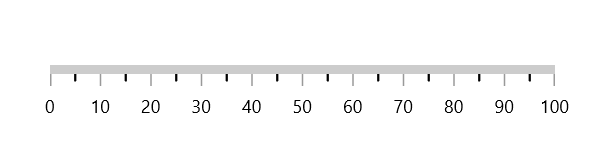### Setting dashed tick lines

By defining `StrokeDashArray` property in both the `MajorTickStyle` and `MinorTickStyle`, you can draw the dashed tick line.

``````<Page.Resources>
<Style x:Key="MajorTickLineStyle"
TargetType="Line">
<Setter Property="Stroke"
Value="Black"></Setter>
<Setter Property="StrokeDashArray"
Value="5,2.5"></Setter>
</Style>

<Style x:Key="MinorTickLineStyle"
TargetType="Line">
<Setter Property="Stroke"
Value="Black"></Setter>
<Setter Property="StrokeDashArray"
Value="3,2.5"></Setter>
</Style>
</Page.Resources>

<gauge:SfLinearGauge>
<gauge:SfLinearGauge.Axis>
<gauge:LinearAxis MajorTickLength="15"
MinorTickLength="10"
MajorTickStyle="{StaticResource MajorTickLineStyle}"
MinorTickStyle="{StaticResource MinorTickLineStyle}" />
</gauge:SfLinearGauge.Axis>
</gauge:SfLinearGauge>``````
``````SfLinearGauge sfLinearGauge = new SfLinearGauge();
sfLinearGauge.Axis.MajorTickLength = 15;
sfLinearGauge.Axis.MinorTickLength = 10;
sfLinearGauge.Axis.MajorTickStyle = this.Resources["MajorTickLineStyle"] as Style;
sfLinearGauge.Axis.MinorTickStyle = this.Resources["MinorTickLineStyle"] as Style;
this.Content = sfLinearGauge;``````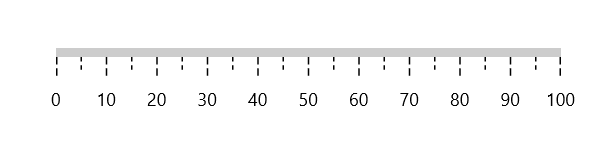### Setting minor ticks count

The major ticks are generated based on the `Interval` property. Similarly, the minor ticks are generated using the `MinorTicksPerInterval` property of axis. By default, the count value of this property is 1.

``````<gauge:SfLinearGauge>
<gauge:SfLinearGauge.Axis>
<gauge:LinearAxis MinorTicksPerInterval="4" />
</gauge:SfLinearGauge.Axis>
</gauge:SfLinearGauge>``````
``````SfLinearGauge sfLinearGauge = new SfLinearGauge();
sfLinearGauge.Axis.MinorTicksPerInterval = 4;
this.Content = sfLinearGauge;``````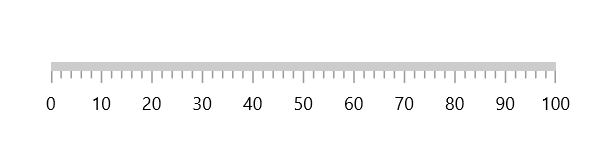### Tick line visibility

The `ShowTicks` property of the axis can be used to enable or disable the visibility of both the major and the minor ticks of axis.

``````<gauge:SfLinearGauge>
<gauge:SfLinearGauge.Axis>
<gauge:LinearAxis ShowTicks="False" />
</gauge:SfLinearGauge.Axis>
</gauge:SfLinearGauge>``````
``````SfLinearGauge sfLinearGauge = new SfLinearGauge();
sfLinearGauge.Axis.ShowTicks = false;
this.Content = sfLinearGauge;``````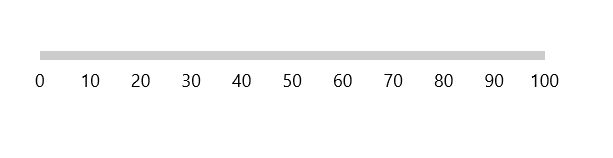### Tick placement

The `Linear axis` allows to position the ticks either inside or outside or center of the axis line using the `TickPosition` property. By default, ticks are positioned inside the axis line.

``````<gauge:SfLinearGauge>
<gauge:SfLinearGauge.Axis>
<gauge:LinearAxis TickPosition="Outside" />
</gauge:SfLinearGauge.Axis>
</gauge:SfLinearGauge>``````
``````SfLinearGauge sfLinearGauge = new SfLinearGauge();
sfLinearGauge.Axis.LabelPosition = GaugeLabelsPosition.Outside;
this.Content = sfLinearGauge;``````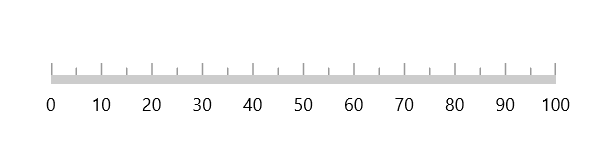### Tick position customization

The ticks can be moved near or far to the axis line using the `TickOffset` property. The default value of `TickOffset` is 0.

``````<gauge:SfLinearGauge>
<gauge:SfLinearGauge.Axis>
<gauge:LinearAxis TickOffset="50" />
</gauge:SfLinearGauge.Axis>
</gauge:SfLinearGauge>``````
``````SfLinearGauge sfLinearGauge = new SfLinearGauge();
sfLinearGauge.Axis.TickOffset = 50;
this.Content = sfLinearGauge;``````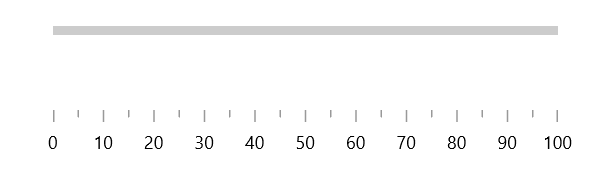## Custom scale range

`Linear gauge` allows you to define a custom scale range by extending the axis based on your business logic.

``````<gauge:SfLinearGauge>
<gauge:SfLinearGauge.Axis>
<local:LogarithmicAxis Minimum="1"
Maximum="10000" />
</gauge:SfLinearGauge.Axis>
</gauge:SfLinearGauge>``````
``````public class LogarithmicAxis : LinearAxis
{
int labelsCount;

public override List<AxisLabelData> GenerateVisibleLabels()
{
List<AxisLabelData> labelInfos = new List<AxisLabelData>();
int minimum = (int)LogBase(this.Minimum, 10);
int maximum = (int)LogBase(this.Maximum, 10);
for (int i = minimum; i <= maximum; i++)
{
double value = Math.Floor(Math.Pow(10, i)); // logBase  value is 10
AxisLabelData label = new AxisLabelData()
{
Value = value,
Text = value.ToString()
};
}

labelsCount = labelInfos.Count;
return labelInfos;
}

private double LogBase(double value, int baseValue)
{
return Math.Log(value) / Math.Log(baseValue);
}

public override double ValueToFactor(double value)
{
return LogBase(value, 10) / (labelsCount - 1);
}

public override double FactorToValue(double factor)
{
return Math.Pow(Math.E, factor * (labelsCount - 1) * Math.Log(10));
}
}``````